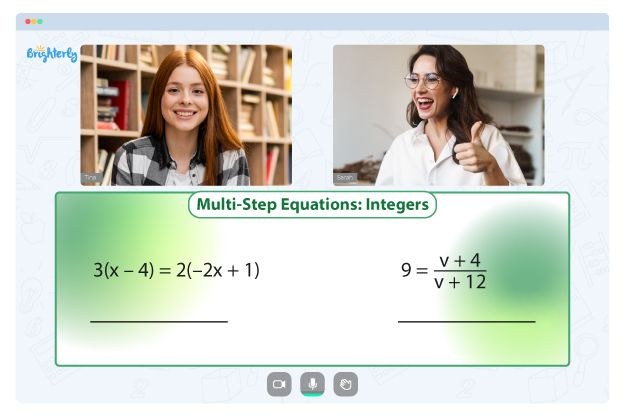# Regrouping in Math – Definition With Examples

Welcome to Brighterly, your go-to resource for making math fun and accessible for children! In this article, we will dive into the concept of regrouping in math. We’ll provide a clear definition of regrouping and offer some engaging examples to help young learners grasp this fundamental concept. So, let’s get started!

## What is Regrouping in Math?

Regrouping, also known as carrying or borrowing, is a mathematical process used when adding or subtracting numbers with multiple digits. It involves rearranging the digits in a column to accurately perform calculations. Regrouping is an essential skill that enables us to work with larger numbers efficiently and accurately.

## How Does Regrouping Work?

To understand regrouping, let’s consider an addition example. Imagine we want to add 27 and 48 together. When we add the units column (7 + 8), we get 15. In this case, we cannot write down the entire sum of 15 in the units column, as it is a two-digit number. Instead, we write down the digit 5 and carry over the digit 1 to the tens column. This process of carrying over is known as regrouping.

Now, let’s add the tens column (2 + 4) along with the carried-over digit of 1. We get a sum of 7. Therefore, the final result of 27 + 48 is 75. Regrouping ensures that we accurately combine the digits and maintain the correct place values.

## Regrouping in Subtraction

Regrouping is not limited to addition; it is equally important in subtraction. Let’s explore a subtraction example to understand regrouping in this context. Consider the subtraction problem 63 – 29. When we subtract the units column (3 – 9), we encounter a situation where the top digit is smaller than the bottom digit. In such cases, regrouping is required.

To regroup, we borrow 1 from the tens column and add it to the units column, making the bottom digit 13 instead of 3. Now we can subtract 13 – 9, resulting in 4. Next, we subtract the tens column (6 – 2), which gives us 4. Hence, the solution to 63 – 29 is 34.

## Why is Regrouping Important?

Regrouping lays the foundation for more advanced mathematical operations, such as multiplication and long division. It helps children develop a deeper understanding of place value and the relationships between digits in a number. By mastering regrouping, young learners gain confidence in working with larger numbers and solving complex math problems.

## Examples of Regrouping in Math

To further illustrate the concept of regrouping, let’s explore some examples:

1. Start by adding the units column: 6 + 8 = 14. Write down 4 in the units column and carry over 1 to the tens column.
2. Move to the tens column: 1 (carried over) + 5 + 3 = 9. Write down 9 in the tens column.

The sum of 56 and 38 is 94.

### Example 2: Subtraction

Problem: Subtract 543 from 900.

1. Begin by subtracting the units column: 0 (borrowed from the tens column) – 3 = 7.
2. Move to the tens column: 0 (borrowed from the hundreds column) – 4 = 6.
3. Finally, subtract the hundreds column: 8 – 5 = 3.

The difference between 900 and 543 is 357.

## Conclusion

Regrouping is a vital concept in mathematics that enables us to accurately perform calculations with multi-digit numbers. Whether it’s addition or subtraction, regrouping plays a crucial role in maintaining the correct place values and ensuring accurate results. By practicing regrouping, children enhance their math skills and prepare themselves for more advanced mathematical concepts.

We hope this article has shed light on the definition and importance of regrouping in math. If you’re looking for more educational resources, be sure to explore the wide range of math activities and games available on Brighterly.

### Why is regrouping sometimes called carrying or borrowing?

Regrouping is often referred to as carrying or borrowing because it involves moving digits from one column to another during addition or subtraction. When we carry over a digit from a smaller place value column to a larger one, it is called carrying. Conversely, when we borrow a digit from a larger place value column to a smaller one, it is called borrowing.

### At what grade level is regrouping typically introduced?

Regrouping is typically introduced in the early elementary grades, around second or third grade. It builds upon the foundational understanding of place value and helps students develop fluency in arithmetic operations with multi-digit numbers.

### Are there any alternative methods to regrouping?

Yes, there are alternative methods to regrouping, such as using manipulatives like base-ten blocks or employing mental math strategies. These alternative approaches can be useful for reinforcing the concept of regrouping before transitioning to traditional written algorithms.

### How can I help my child practice regrouping at home?

You can engage your child in various hands-on activities and games to practice regrouping. Utilize visual aids, such as place value charts or manipulatives, to reinforce the concept. Additionally, online math resources and interactive games can provide an enjoyable way for children to practice regrouping skills.

### Is regrouping only applicable to addition and subtraction?

While regrouping is primarily associated with addition and subtraction, it is a fundamental concept that extends to other mathematical operations. Regrouping is also essential in multiplication (e.g., carrying over digits in the multiplication algorithm) and division (e.g., borrowing when dividing).

Sources:

Problems with Equations?• Is your child having trouble understanding equations fundamentals?
• An online tutor could provide the necessary assistance.

Does your child need extra support with understanding equations lessons? Start lessons with an online tutor.